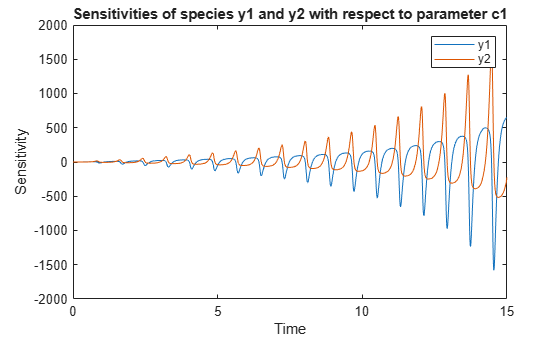# getsensmatrix

Get 3-D sensitivity matrix from `SimData` object

## Syntax

``[t,r,outputFactors,inputFactors] = getsensmatrix(simdata)``
``[t,r,outputFactors,inputFactors] = getsensmatrix(simdata,outputFactorNames,inputFactorNames)``

## Description

example

````[t,r,outputFactors,inputFactors] = getsensmatrix(simdata)` returns the time `t` and sensitivity data `r` as well as all the `outputFactors` and `inputFactors` (sensitivity outputs and inputs) from the `SimData` object `simdata`.```

example

````[t,r,outputFactors,inputFactors] = getsensmatrix(simdata,outputFactorNames,inputFactorNames)` returns the sensitivity data for only the outputs and inputs specified by `outputFactorNames` and `inputFactorNames`, respectively.```

## Examples

collapse all

This example shows how to calculate the local sensitivities of some species in the Lotka-Volterra model using the `SimFunctionSensitivity` object.

Load the sample project.

`sbioloadproject lotka;`

Define the input parameters.

`params = {'Reaction1.c1', 'Reaction2.c2'};`

Define the observed species, which are the outputs of simulation.

`observables = {'y1', 'y2'};`

Create a `SimFunctionSensitivity` object. Set the sensitivity output factors to all species (`y1` and `y2`) specified in the `observables` argument and input factors to those in the `params` argument (`c1` and `c2`) by setting the name-value pair argument to `'all'`.

`f = createSimFunction(m1,params,observables,[],'SensitivityOutputs','all','SensitivityInputs','all','SensitivityNormalization','Full')`
```f = SimFunction Parameters: Name Value Type ________________ _____ _____________ {'Reaction1.c1'} 10 {'parameter'} {'Reaction2.c2'} 0.01 {'parameter'} Observables: Name Type ______ ___________ {'y1'} {'species'} {'y2'} {'species'} Dosed: None Sensitivity Input Factors: Name Type ________________ _____________ {'Reaction1.c1'} {'parameter'} {'Reaction2.c2'} {'parameter'} Sensitivity Output Factors: Name Type ______ ___________ {'y1'} {'species'} {'y2'} {'species'} Sensitivity Normalization: Full ```

Calculate sensitivities by executing the object with `c1` and `c2` set to 10 and 0.1, respectively. Set the output times from 1 to 10. `t` contains time points, `y` contains simulation data, and `sensMatrix` is the sensitivity matrix containing sensitivities of `y1` and `y2` with respect to `c1` and `c2`.

`[t,y,sensMatrix] = f([10,0.1],[],[],1:10);`

Retrieve the sensitivity information at time point 5.

```temp = sensMatrix{:}; sensMatrix2 = temp(t{:}==5,:,:); sensMatrix2 = squeeze(sensMatrix2)```
```sensMatrix2 = 2×2 37.6987 -6.8447 -40.2791 5.8225 ```

The rows of `sensMatrix2` represent the output factors (`y1` and `y2`). The columns represent the input factors (`c1` and `c2`).

`$sensMatrix2=\left[\begin{array}{cc}\begin{array}{c}\frac{\partial y1}{\partial c1}\\ \\ \frac{\partial y2}{\partial c1}\end{array}& \begin{array}{c}\frac{\partial y1}{\partial c2}\\ \\ \frac{\partial y2}{\partial c2}\end{array}\end{array}\right]$`

Set the stop time to 15, without specifying the output times. In this case, the output times are the solver time points by default.

`sd = f([10,0.1],15);`

Retrieve the calculated sensitivities from the `SimData` object `sd`.

`[t,y,outputs,inputs] = getsensmatrix(sd);`

Plot the sensitivities of species `y1` and `y2` with respect to `c1`.

```figure; plot(t,y(:,:,1)); legend(outputs); title('Sensitivities of species y1 and y2 with respect to parameter c1'); xlabel('Time'); ylabel('Sensitivity');```Plot the sensitivities of species `y1` and `y2` with respect to `c2`.

```figure; plot(t,y(:,:,2)); legend(outputs); title('Sensitivities of species y1 and y2 with respect to parameter c2'); xlabel('Time'); ylabel('Sensitivity');```Alternatively, you can use `sbioplot`.

`sbioplot(sd);`You can also plot the sensitivity matrix using the time integral for the calculated sensitivities of `y1` and `y2`. The plot indicates `y1` and `y2` are more sensitive to `c1` than `c2`.

```[~, in, out] = size(y); result = zeros(in, out); for i = 1:in for j = 1:out result(i,j) = trapz(t(:),abs(y(:,i,j))); end end figure; hbar = bar(result); haxes = hbar(1).Parent; haxes.XTick = 1:length(outputs); haxes.XTickLabel = outputs; legend(inputs,'Location','NorthEastOutside'); ylabel('Sensitivity');```## Input Arguments

collapse all

Simulation data, specified as a `SimData` object or array of `SimData` objects. If `simdata` is an array of objects, the outputs are cell arrays in which each cell contains data for the corresponding object in the `SimData` array.

Names of sensitivity outputs, specified as an empty array `[]`, character vector, string, string vector, or cell array of character vectors.

By default, the function uses an empty array `[]` to return sensitivity data for all output factors in `simdata`.

Names of sensitivity inputs, specified as an empty array `[]`, character vector, string, string vector, or cell array of character vectors.

By default, the function uses an empty array `[]` to return sensitivity data on all input factors in `simdata`.

## Output Arguments

collapse all

Simulation time points for the sensitivity data, returned as an m-by-1 numeric vector or cell array. m is the number of time points.

Sensitivity data, returned as an m-by-n-by-p array or cell array. m is the number of time points, n is the number of sensitivity outputs, and p is the number of sensitivity inputs.

The `outputFactors` output argument labels the second dimension of `r` and `inputFactors` labels the third dimension of `r`. For example, `r(:,i,j)` is the time course for the sensitivity of the state `outputFactors{i}` to the input `inputFactor{j}`.

The function returns only the sensitivity data already in the `SimData` object. It does not calculate the sensitivities. For details on setting up and performing a sensitivity calculation, see Local Sensitivity Analysis (LSA). During setup, you can also specify how to normalize the sensitivity data.

Names of sensitivity outputs, returned as an n-by-1 cell array. n is the number of sensitivity outputs.

The output factors are the states for which you calculated the sensitivities. In other words, the sensitivity outputs are the numerators. For more information, see Local Sensitivity Analysis (LSA).

Names of sensitivity inputs, returned as an p-by-1 cell array. p is the number of input factors.

The input factors are the states with respect to which you calculated the sensitivities. In other words, the sensivity inputs are the denominators as explained in Local Sensitivity Analysis (LSA).

## Version History

Introduced in R2008b